Next: *MASS Up: Input deck format Previous: *KINEMATIC   Contents

## *MAGNETIC PERMEABILITY

Keyword type: model definition, material

This option is used to define the magnetic permeability of a material. There are no parameters. The material is supposed to be isotropic. The constant may be temperature dependent. The unit of the magnetic permeability coefficient is the unit of force divided by the square of the unit of electrical current. In SI-units this is N/A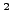or Henry/m. The magnetic permeability may be viewed as the product of the relative magnetic permeability (dimensionless)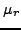with the permeability of vacuum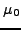.

Following the magnetic permeability constant the user is supposed to define the domain for which this material is used. In an electromagnetic calculation there are three domains:

1. the air, which is the P-domain (domain 1)
2. the bodies, which is the A,V-domain (domain 2)
3. that part of the air, which, if filled with body material, ensures that the bodies are simply connected; this is the A-domain (domain 3). If there is only one body and if it is such that it is naturally simply connected the A-domain is empty.

For more details the reader is referred to the section on electromagnetism.

First line:

• *MAGNETIC PERMEABILITY

Following line:

•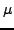.
• number of the domain
• Temperature.
Repeat this line if needed to define complete temperature dependence.

Example:

*MAGNETIC PERMEABILITY
1.255987E-6,2


tells you that the magnetic permeability coefficient is 1.255987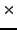10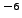, independent of temperature (if SI-units are used this is the magnetic permeability of copper). The domain for which this material is defined is the A,V-domain.

Example files: induction.Next: *MASS Up: Input deck format Previous: *KINEMATIC   Contents
guido dhondt 2018-12-15